ggplot2 is a plotting system for R created by Hadley Wickham. ggplot2 offers a consistent and systematic approach to generate graphics based on the book Grammar of Graphics by Leland Wilkinson (data, aesthetic mapping, geoms, stats, scales, facets and themes). ggplot2 takes care of many of the fiddly details that make plotting a hassle (like drawing legends) as well as providing a powerful model of graphics that makes it easy to produce complex multi-layered graphics. However, it is a comprehensive system and thus, not as easy to learn. Visit the ggplot2 website or take a quick tour through the R graph gallery featuring ggplot2 to get an overview of the functionality provided by ggplot2. Further, the Cookbook for R is a valuable source, as it provides solutions to common tasks and problems in analyzing data. Moreover, RStudio provides a quite helpful cheat sheet summarizing the main ggplot2 functions.

Please be aware that within the scope of this tutorial we will only scratch the surface of the functionality of ggplot2. However, we highly recommend to apply ggplot2 to as many as possible of the plotting tasks you are facing. You will learn to master the grammar of graphics step by step and finally you may leverage the full power of this awesome plotting library.

The grammar of graphics with ggplot2

ggplot2 is based on the grammar of graphics. The basic idea of grammar of graphics, and hence of ggplot2 is, that you can build every graph from the same few components:

• a data set,

• a set of geom’s: visual marks that represent data points, and

• a coordinate system. In addition there are some more components, such as statistical transformation (stat), scales, position adjustments and faceting, to allow fine tuning and enhance the spectrum of graphs being generated.

In order to begin a plot we apply the ggplot() function. The plot is finished by adding layers using the + operator.

ggplot(data, aes(x, y))

To display data values, variables in the data set are mapped (aesthetic mapping = “something you can see”) to aesthetic properties of the geom like size, color, shape, and x and y locations.

Note that there is a helper function called qplot() (for quick plot) that can hide much of this complexity when creating standard graphs.

Once again, we make use of the students data set. You may download the students.csv file here.

students <- read.csv("https://userpage.fu-berlin.de/soga/data/raw-data/students.csv")

For a less packed visualization we restrict the data set to the first 100 entries.

students100 <- students[1:100, ]

Let us construct a ggplot2 plot layer. We start with a scatter plot of the variables weight and height.

First we load the library ggplot2. If you have not installed ggplot2 type install.packages("ggplot2") into your console.

library(ggplot2)

We initialize the plot using the ggplot() function and add a geom, more specifically a geom_point() (hence, we want points to be plotted). We use aes() to specify the variables in the data to be mapped as visual properties (aesthetics) of the geometric object (geom_point()).

p <- ggplot(
data = students100,
aes(x = weight, y = height)
) +
geom_point()
p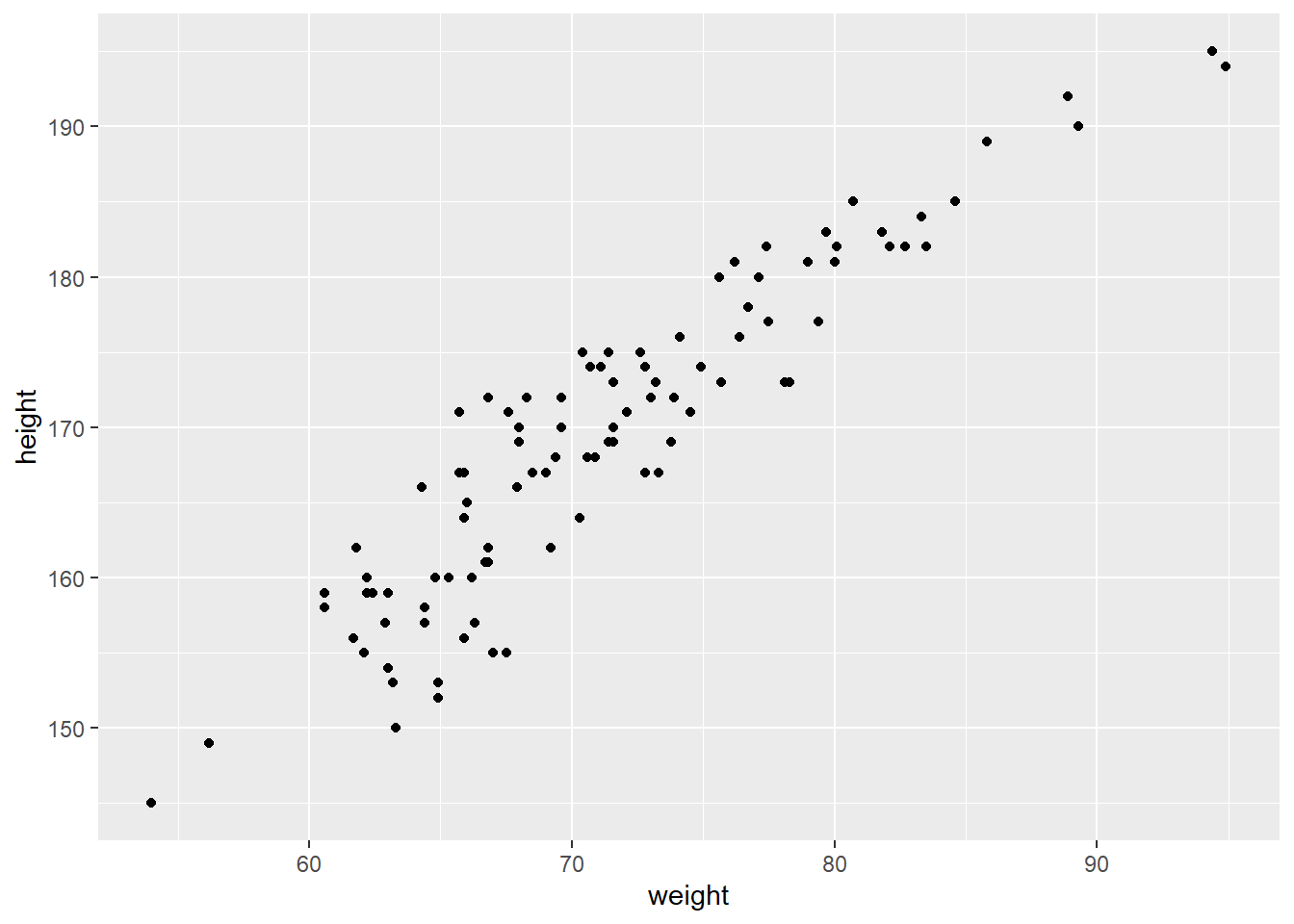Just three lines of code and we get back a quite nice looking plot!

In addition, there are several aesthetic properties available, such as color, shape and size. By adding color = gender or shape = gender to the aes() function we can condition the data on the categorical variable gender.

ggplot(
data = students100,
aes(x = weight, y = height)
) +
geom_point(aes(color = gender))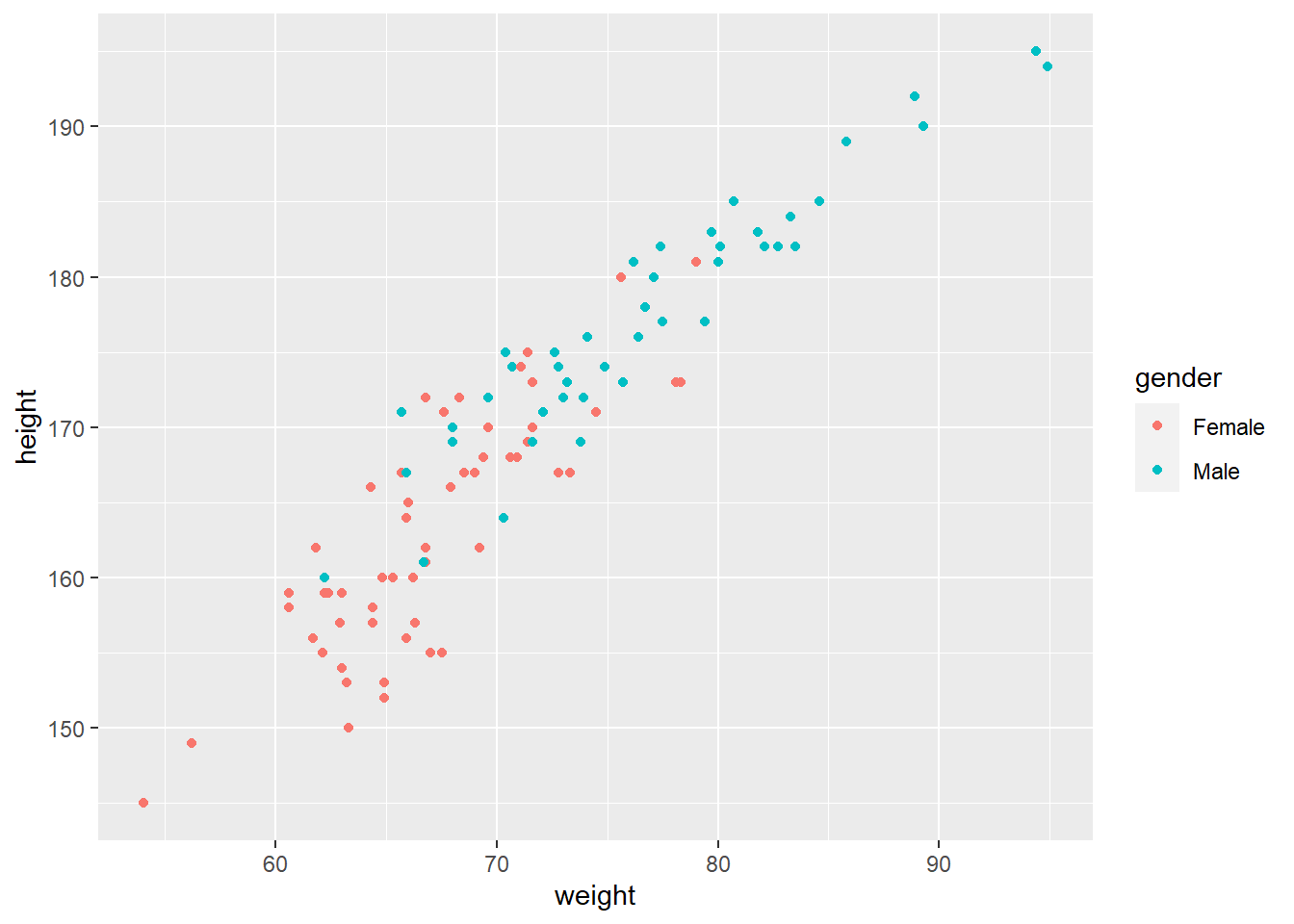ggplot(
data = students100,
aes(x = weight, y = height)
) +
geom_point(aes(shape = gender))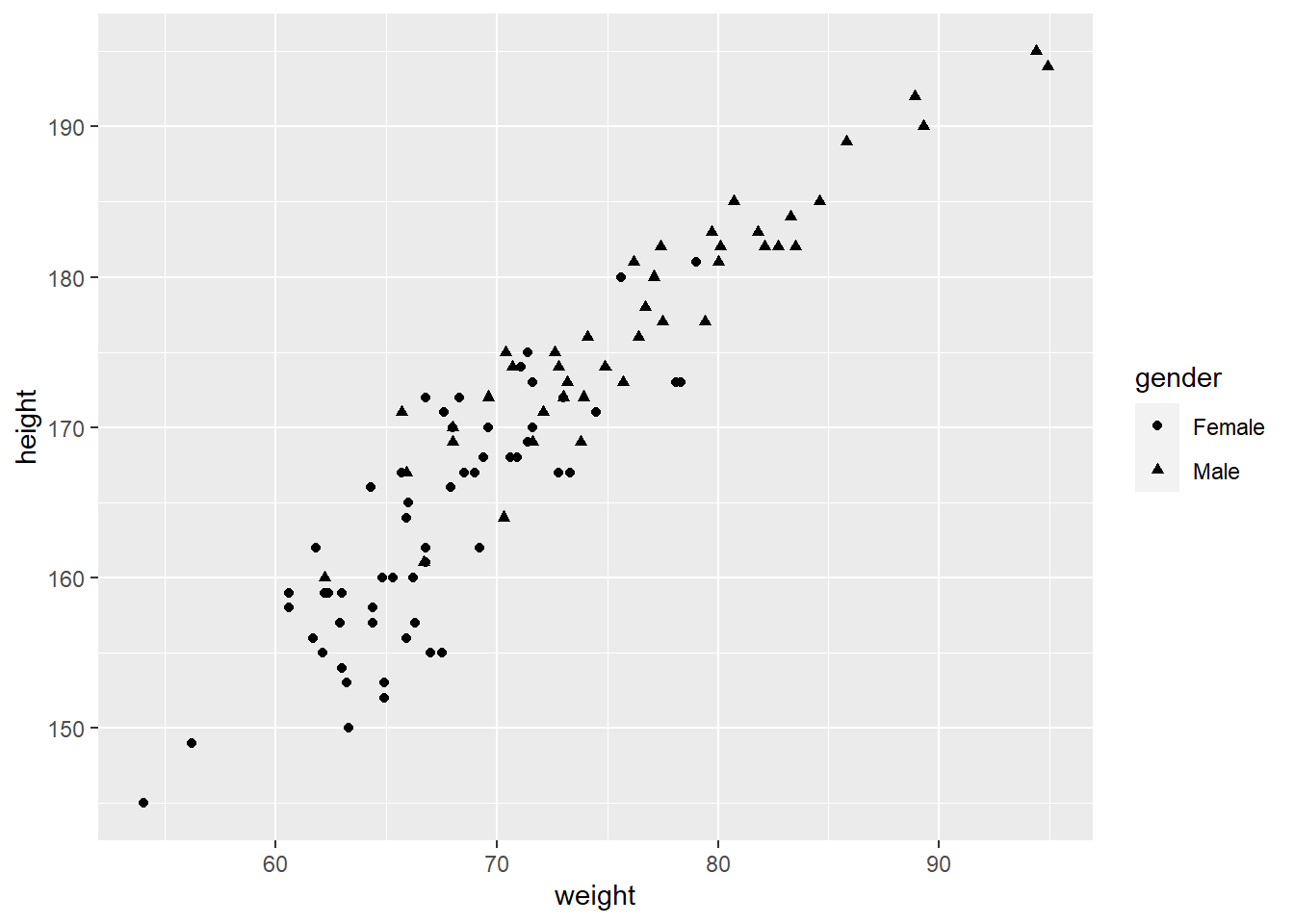We may easily expand this approach and add size = age to the aes() function in order to relate the size of the points to the age of the particular student. Please note how ggplot2 takes care of adding a nice looking legend to the graph. Finally, we assign our graph the variable p to save it for further processing.

p <- ggplot(
data = students100,
aes(x = weight, y = height)
) +
geom_point(aes(size = age, color = gender))
p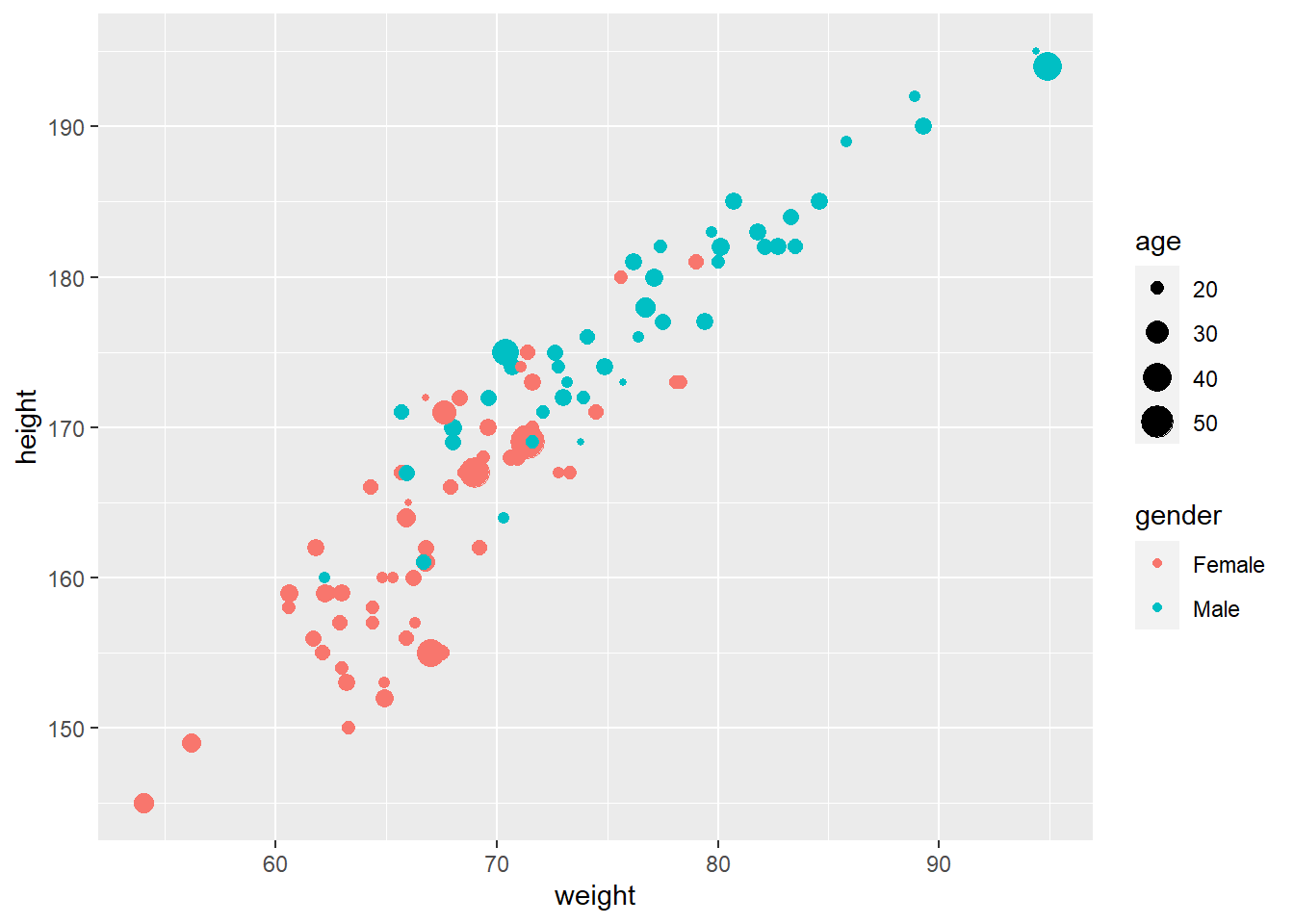We can also apply more than one geom to our graph. For this, we now add geom_smooth() to our graph p defined above. We use method = 'loess' as a property (lm, linear model is the default) to add a locally weighted scatter plot smoothing. Note that by default the standard error band se = TRUE will be plotted. We can avoid this behavior by setting se = FALSE.

p <- p +
geom_smooth(
method = "loess"
# , se = FALSE   ## uncomment to avoid plotting CI
)
p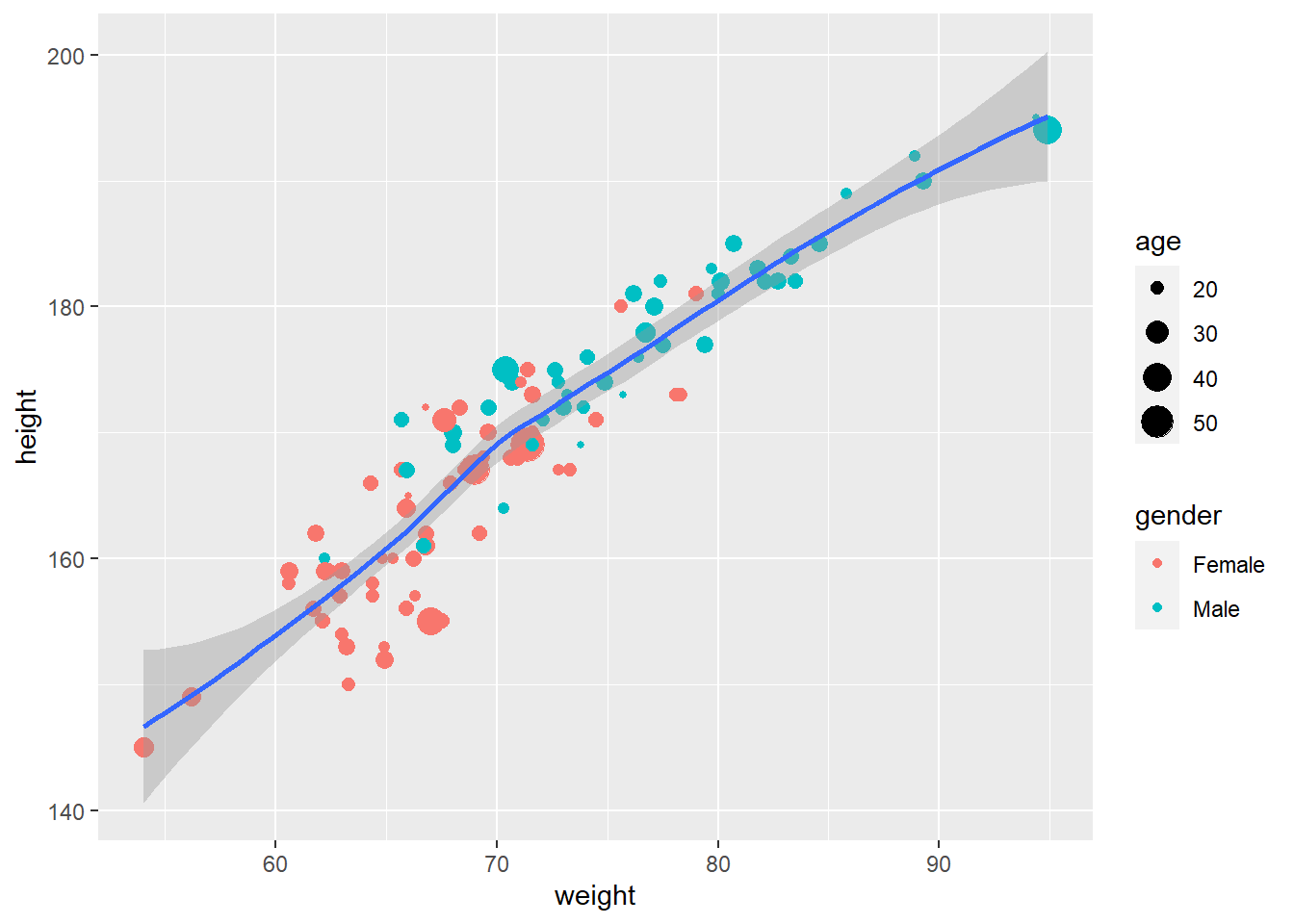Finally, we add a title (ggtitle()) to the graph and change the theme (theme_bw()) to be a white background with grid lines.

p <- p +
ggtitle("my first graph with ggplot2") +
theme_bw()
p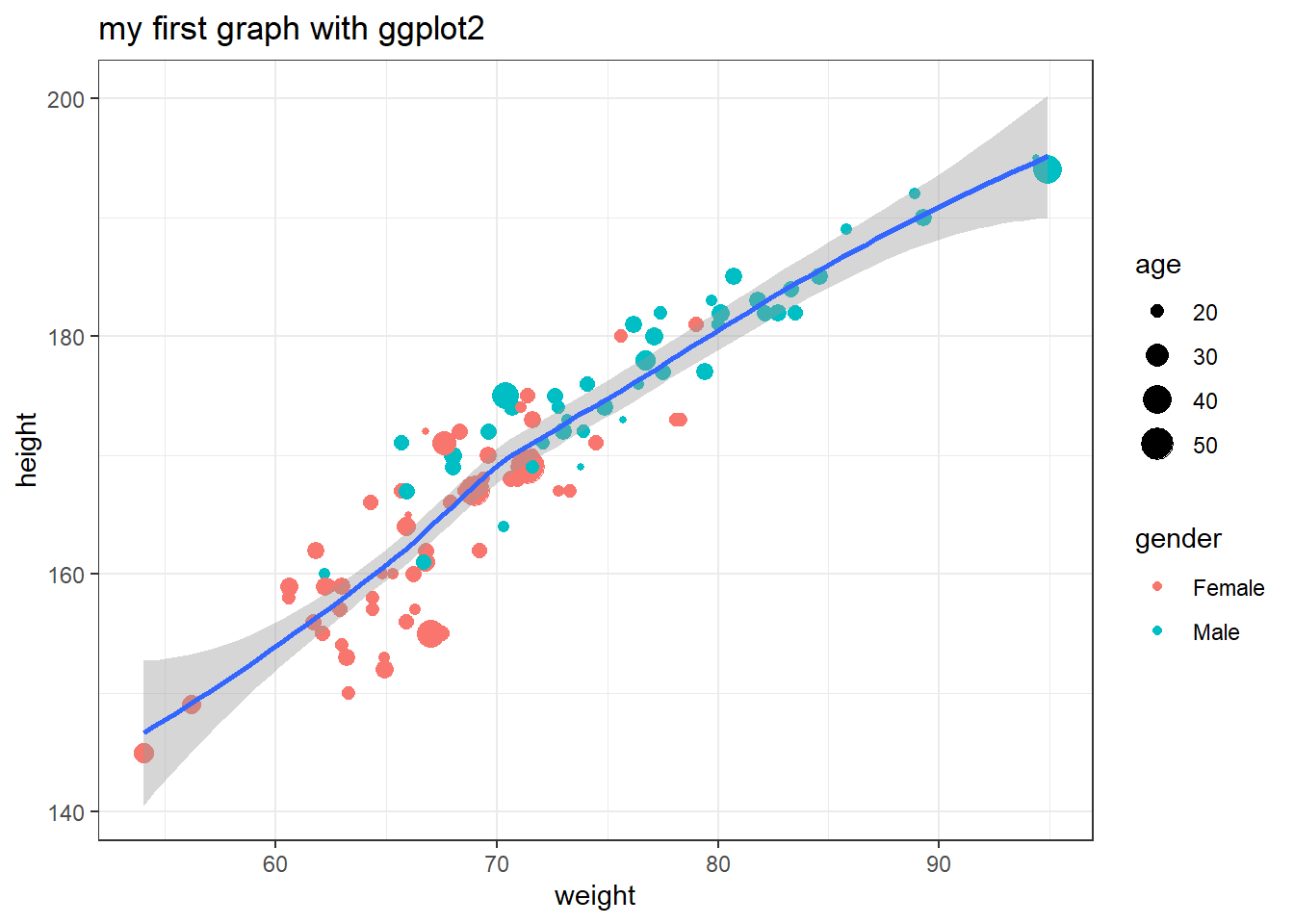To conclude this section we show some more simple graph examples using the ggplot2 package. Hopefully, this quick tour through ggplot2 encouraged you to apply this plotting library for your own tasks.

Boxplot

ggplot(
data = students100,
aes(x = religion, y = age)
) +
stat_boxplot() +
geom_point() +
theme_bw()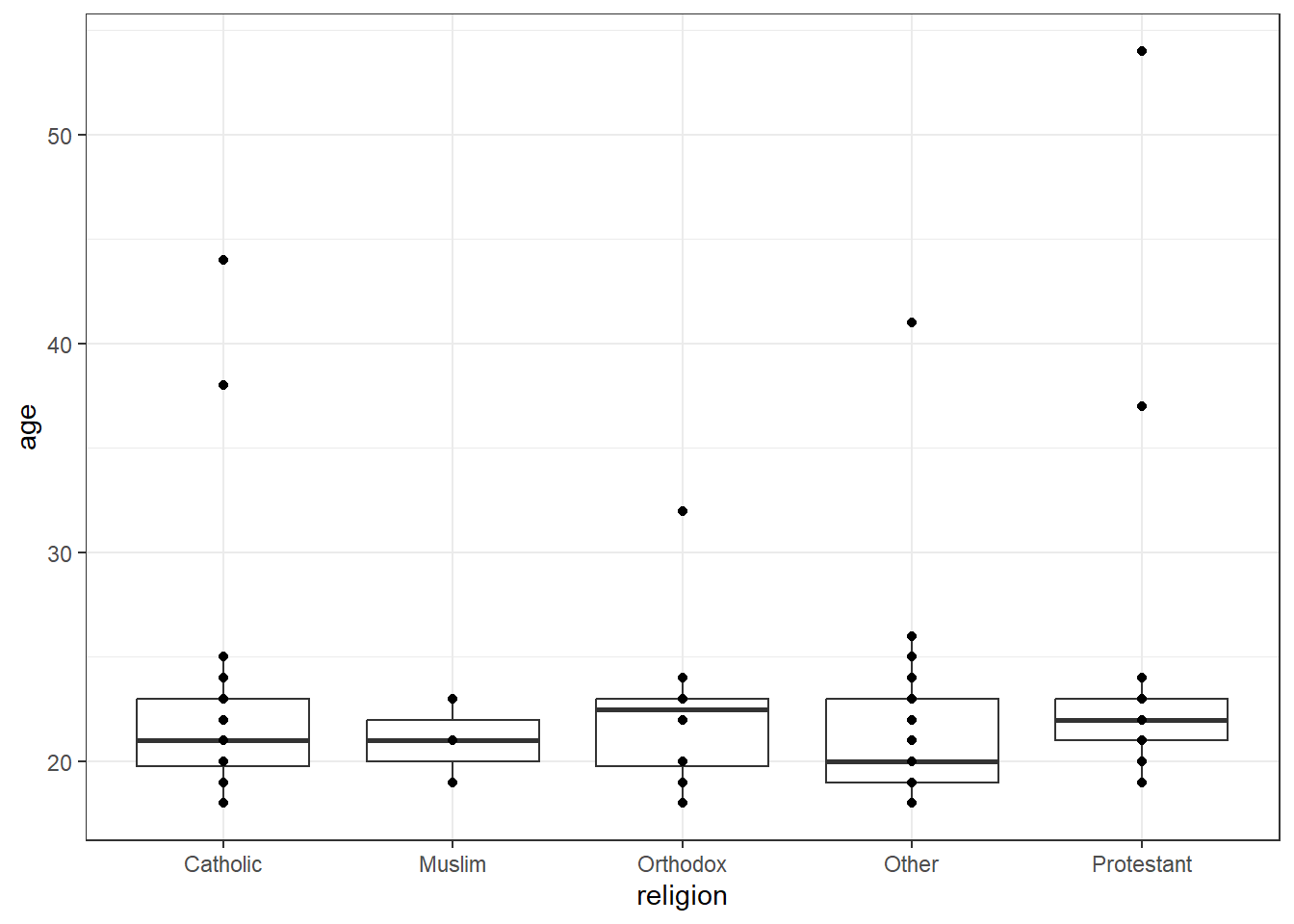Conditioned boxplot

ggplot(
data = students100,
aes(x = religion, y = age)
) +
stat_boxplot(aes(fill = religion)) +
geom_point() +
facet_wrap(~gender) +
theme_bw()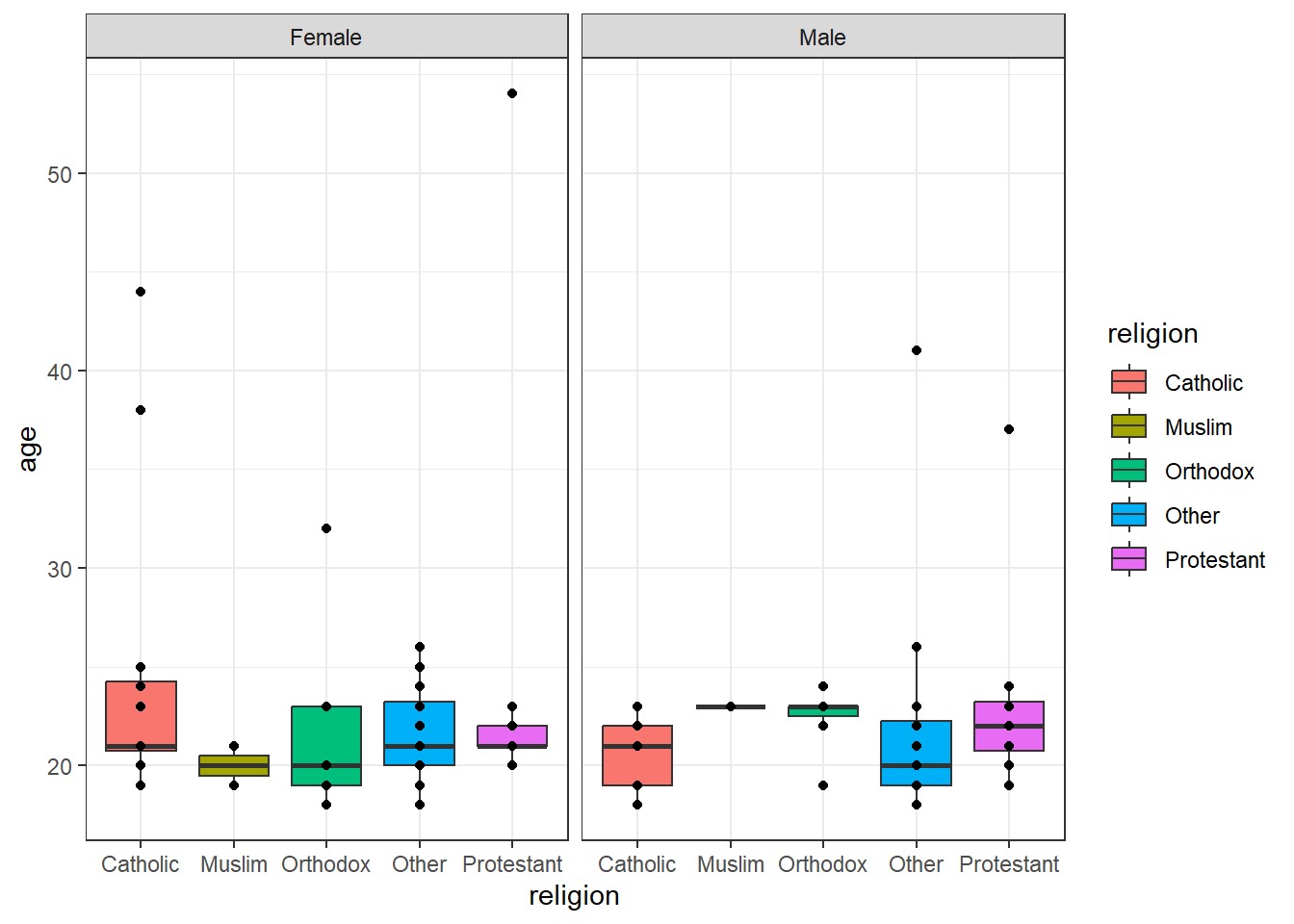Barplot

ggplot(data = students100, aes(fill = major, y = salary, x = religion)) +
geom_bar(stat = "identity") +
theme_classic()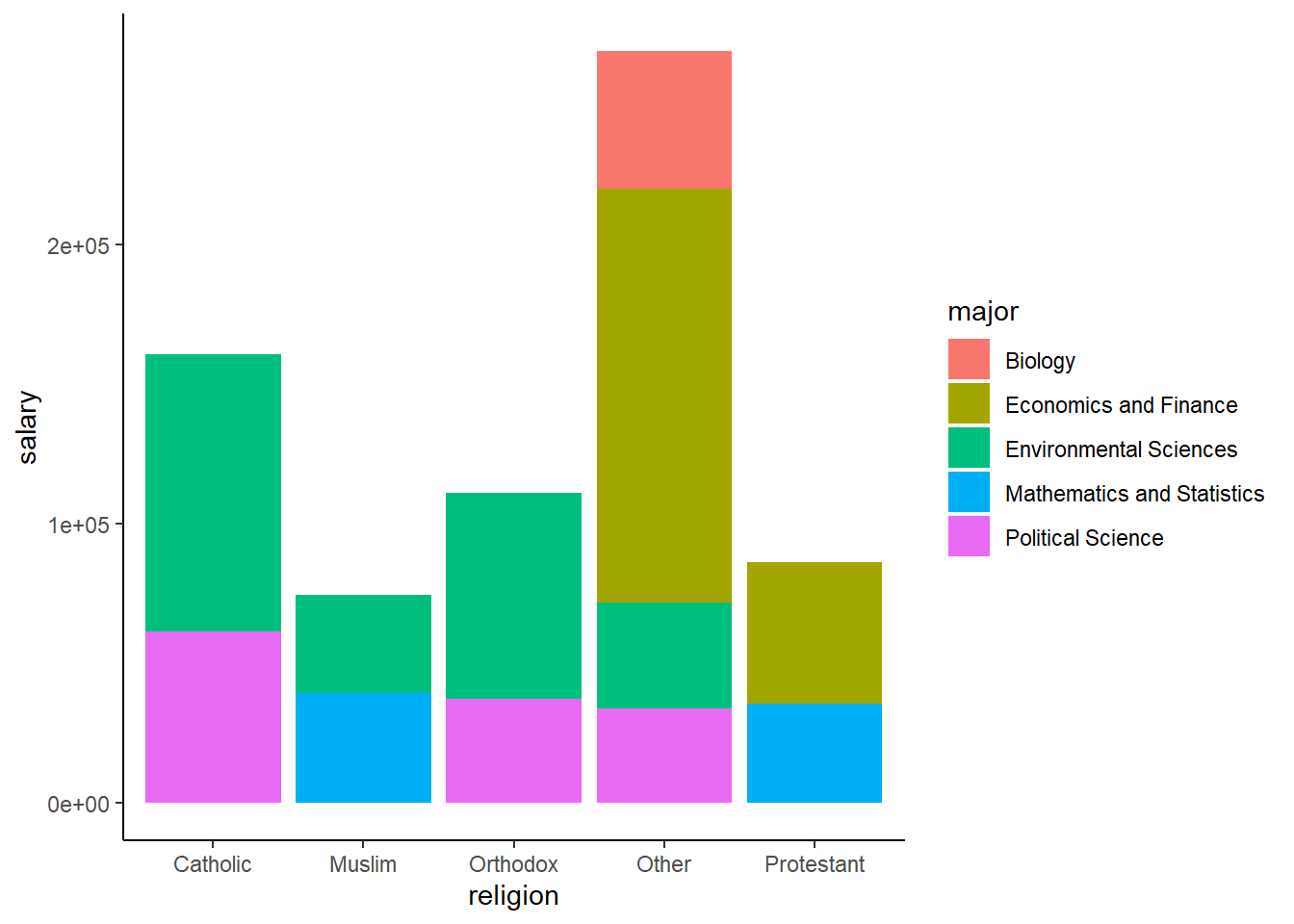Density plot

ggplot(data = students100, aes(weight, colour = gender, fill = gender)) +
geom_density(alpha = 0.55) +
theme_minimal()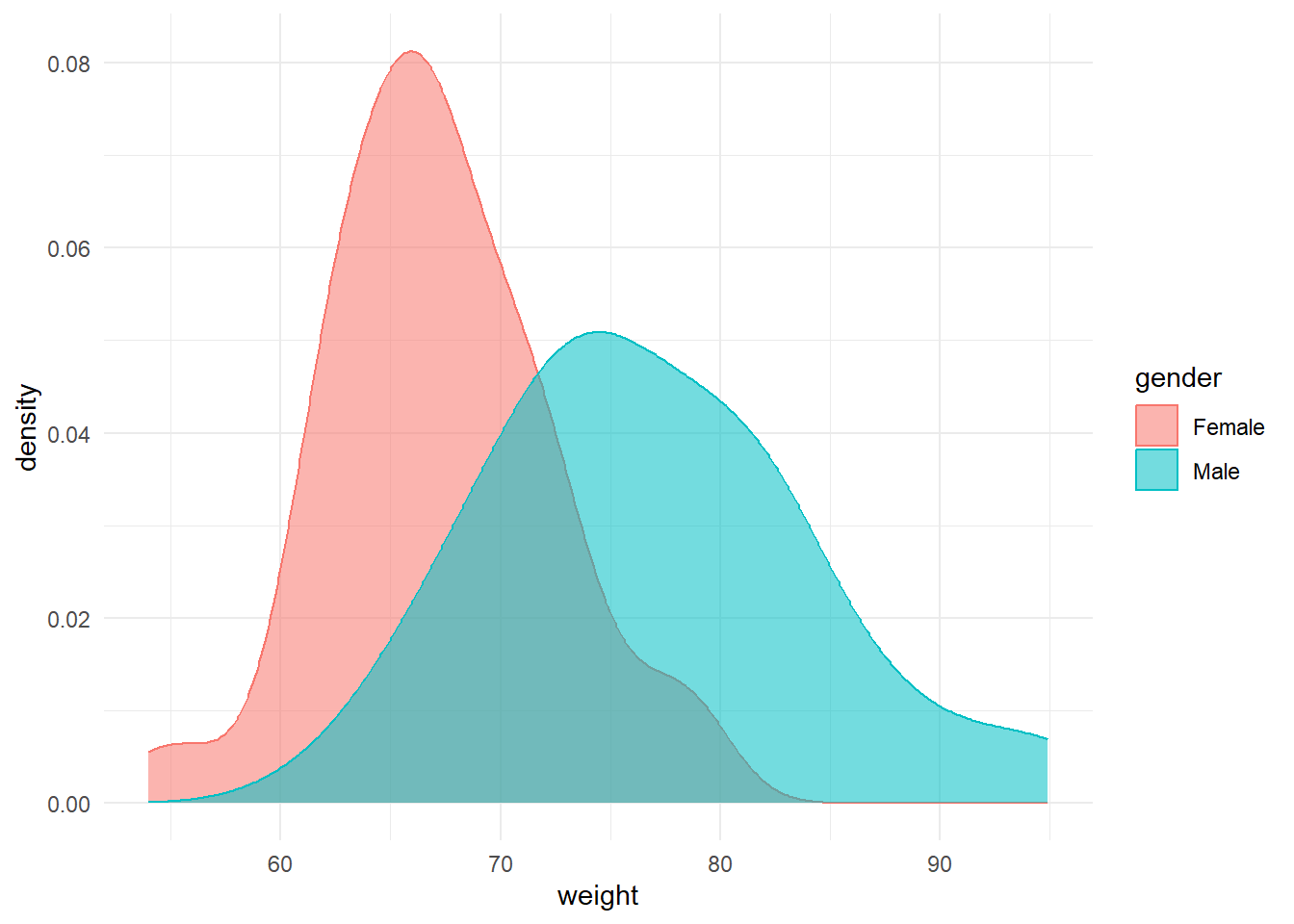Citation

The E-Learning project SOGA-R was developed at the Department of Earth Sciences by Kai Hartmann, Joachim Krois and Annette Rudolph. You can reach us via mail by soga[at]zedat.fu-berlin.de.You may use this project freely under the Creative Commons Attribution-ShareAlike 4.0 International License.

Please cite as follow: Hartmann, K., Krois, J., Rudolph, A. (2023): Statistics and Geodata Analysis using R (SOGA-R). Department of Earth Sciences, Freie Universitaet Berlin.